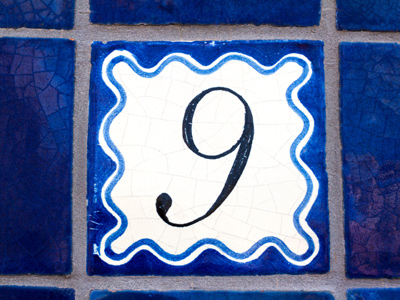How many ones are there in the number 9?

# Level 3-4 Numbers - Place Value

If you want to understand the values of numbers then you'll need to know the values of the places in them. In KS3 Maths you should be well familiar with place values so this quiz will test just how much you've learned.

We know the value of a digit changes with its position in a number relative to the decimal point. Before the decimal we have ones, tens and hundreds, and after we have tenths, hundredths and thousandths. We also use place holders. Zeros at the end of a number are place holders. They come after another number, a 1 for example, to show whether it is worth 10, 100 or 1,000. The number 648.937 is 6 hundreds (600) + 4 tens (40) + 8 ones (8) + 9 tenths + 3 hundredths + 7 thousandths.

The following quiz will recap the subject of place values. Take your time and read each question carefully before choosing your answers. Good luck!

1.
2,468 - re-order this number to get the largest even number.
2,468
4,286
6,842
8,642
All of the digits are even so just re-arrange them - highest, then second highest, then third then least
2.
2,468 - re-order this number to get the smallest odd number.
2,468
4,268
6,284
Not possible
None of the numbers are odd
3.
How many ones are there in the number 9?
5
7
9
11
There are no tens, hundreds or thousands in the number 9
4.
How many thousands are there in the number 4,647?
2
4
6
7
4,000 (thousands) + 600 (hundreds) + 40 (tens) + 7 (ones)
5.
How many hundreds are there in the number 42?
0
1
2
4
The number 42 is lower than 100!
6.
How would you write the number 2,011 in words?
Two hundred, one thousand and one eleven
Two thousand and eleven
Two thousand eleven
Two thousands, no hundreds, one ten and one one
Don't forget the 'and'!
7.
5,221 - re-order this number to get the smallest odd number.
1,225
1,252
1,522
2,512
All the others are even numbers
8.
How many hundreds in the number 6,814?
1
4
6
8
Saying it out loud can be helpful
9.
How would you write the number seven thousand, six hundred and thirty-three in figures?
3,667
6,337
7,366
7,633
7 thousands, 6 hundreds, 3 tens and 3 ones
10.
How many tens are in the number 631?
1
3
6
31
From the right - Ones, Tens, Hundreds